#恒泰-打造专业工程造价咨询公司微信号扫一扫# 商务分怎么算？——探讨服务类项目和EPC类项目在商务评审过程中普遍存在的错误

在招投标实践过程中，很多服务类项目和EPC类项目都是以费率的形式进行报价，细分的话分为两种情况，一种情况是报价就是取费费率，比如设计收费等；另一种情况是报价是优惠幅度，通常也是以费率（优惠率）的方式出现，比如EPC类项目中的施工部分。

EPC类项目中施工部分报价的优惠幅度因各投标人企业不同的管理水平、人员素质、机械设备拥有状况、社会信誉度等情况，导致能够给出的优惠率（费率）也不尽相同，依本人的实践经验和工程造价的普遍规律来看，优惠率一定会控制在合理范围，保证企业能够保证质量的前提下，能有合理的利润空间。基本上最大优惠率没有超过40%（极端情况）的。

EPC类项目中的施工部分：Z=a×S+(1-a)×J

S：各投标人的商务部分得分；

J：各投标人的技术部分得分；

a：权重值，本项目a=0.3

A：30-（2.2-1.79）×1=29.59

B：30-（2-1.79）×1=29.79

C：30-（1.79-1.15）×0.5=29.68

D：30-（1.8-1.79）×1=29.99

A：70-（30-15.7）×0.5=62.85

B：70-（30-30）×0.5=70

C：70-（30-12.5）×0.5=61.25

D：70-（30-18）×0.5=64

A：SA=29.59+62.85=92.44

B：SB=29.79+70=99.79

C：SC=29.68+61.25=90.93

D：SD=29.99+64=93.99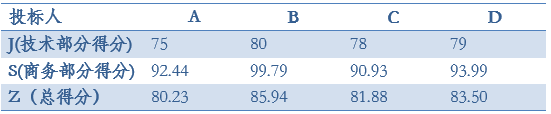A：30-（2.2-1.79）÷1.79×100×1=7.09

B：30-（2-1.79）÷1.79×100×1=18.27

C：30-（1.79-1.15）÷1.79×100×0.5=12.12

D：30-（1.8-1.79）÷1.79×100×1=29.44

A：70-（30-15.7）÷70×100×0.5=59.79

B：70-（30-30）÷70×100×0.5=70

C：70-（30-12.5）÷70×100×0.5=57.5

D：70-（30-18）÷70×100×0.5=61.43

A：SA=7.09+59.79=66.88

B：SB=18.27+70=88.27

C：SC=12.12+57.5=69.62

D：SD=29.44+61.43=90.87A：70-（19.05-15.7）÷80.95×100×1=65.86

B：70-（30-19.05）÷80.95×100×0.5=57.06

C：70-（19.05-12.5）÷80.95×100×1=61.91

D：70-（19.05-18）÷80.95×100×1=68.70

A：SA=7.09+65.86=72.95

B：SB=18.27+57.06=75.33

C：SC=12.12+61.91=74.03

D：SD=29.44+68.70=98.14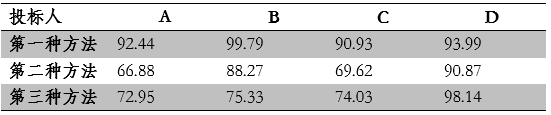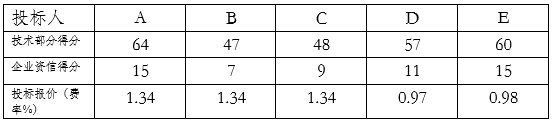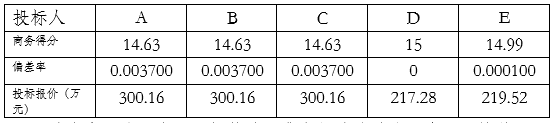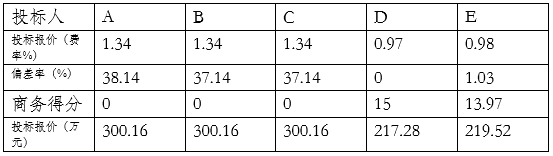2016.02.09